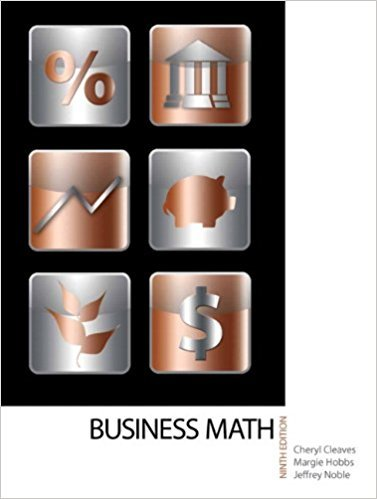×
×

# Solutions for Chapter 16-1: MORTGAGE PAYMENTS## Full solutions for Business Math, | 9th Edition

ISBN: 9780135108178Solutions for Chapter 16-1: MORTGAGE PAYMENTS

Solutions for Chapter 16-1
4 5 0 318 Reviews
27
1
##### ISBN: 9780135108178

Since 14 problems in chapter 16-1: MORTGAGE PAYMENTS have been answered, more than 19273 students have viewed full step-by-step solutions from this chapter. This textbook survival guide was created for the textbook: Business Math, , edition: 9. This expansive textbook survival guide covers the following chapters and their solutions. Chapter 16-1: MORTGAGE PAYMENTS includes 14 full step-by-step solutions. Business Math, was written by and is associated to the ISBN: 9780135108178.

Key Math Terms and definitions covered in this textbook
• Augmented matrix [A b].

Ax = b is solvable when b is in the column space of A; then [A b] has the same rank as A. Elimination on [A b] keeps equations correct.

• Column picture of Ax = b.

The vector b becomes a combination of the columns of A. The system is solvable only when b is in the column space C (A).

• Echelon matrix U.

The first nonzero entry (the pivot) in each row comes in a later column than the pivot in the previous row. All zero rows come last.

• Factorization

A = L U. If elimination takes A to U without row exchanges, then the lower triangular L with multipliers eij (and eii = 1) brings U back to A.

• Incidence matrix of a directed graph.

The m by n edge-node incidence matrix has a row for each edge (node i to node j), with entries -1 and 1 in columns i and j .

• Left nullspace N (AT).

Nullspace of AT = "left nullspace" of A because y T A = OT.

• Linear transformation T.

Each vector V in the input space transforms to T (v) in the output space, and linearity requires T(cv + dw) = c T(v) + d T(w). Examples: Matrix multiplication A v, differentiation and integration in function space.

• Multiplication Ax

= Xl (column 1) + ... + xn(column n) = combination of columns.

• Normal equation AT Ax = ATb.

Gives the least squares solution to Ax = b if A has full rank n (independent columns). The equation says that (columns of A)·(b - Ax) = o.

• Orthonormal vectors q 1 , ... , q n·

Dot products are q T q j = 0 if i =1= j and q T q i = 1. The matrix Q with these orthonormal columns has Q T Q = I. If m = n then Q T = Q -1 and q 1 ' ... , q n is an orthonormal basis for Rn : every v = L (v T q j )q j •

• Partial pivoting.

In each column, choose the largest available pivot to control roundoff; all multipliers have leij I < 1. See condition number.

• Permutation matrix P.

There are n! orders of 1, ... , n. The n! P 's have the rows of I in those orders. P A puts the rows of A in the same order. P is even or odd (det P = 1 or -1) based on the number of row exchanges to reach I.

• Row picture of Ax = b.

Each equation gives a plane in Rn; the planes intersect at x.

• Simplex method for linear programming.

The minimum cost vector x * is found by moving from comer to lower cost comer along the edges of the feasible set (where the constraints Ax = b and x > 0 are satisfied). Minimum cost at a comer!

• Solvable system Ax = b.

The right side b is in the column space of A.

• Special solutions to As = O.

One free variable is Si = 1, other free variables = o.

• Unitary matrix UH = U T = U-I.

Orthonormal columns (complex analog of Q).

v + w = (VI + WI, ... , Vn + Wn ) = diagonal of parallelogram.

• Vector v in Rn.

Sequence of n real numbers v = (VI, ... , Vn) = point in Rn.

• Wavelets Wjk(t).

Stretch and shift the time axis to create Wjk(t) = woo(2j t - k).

×